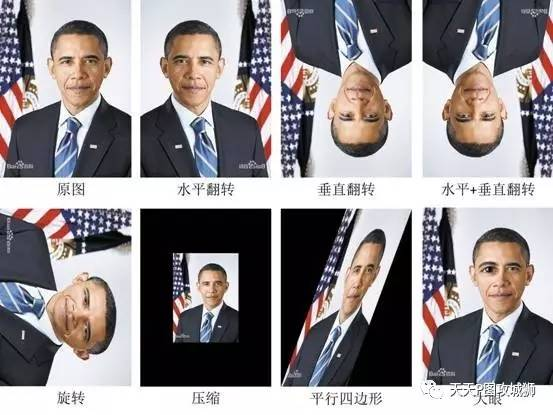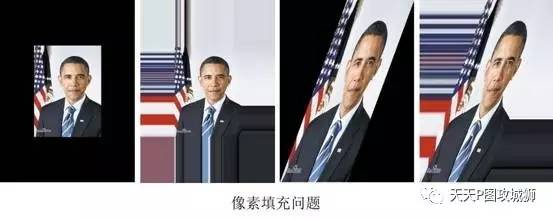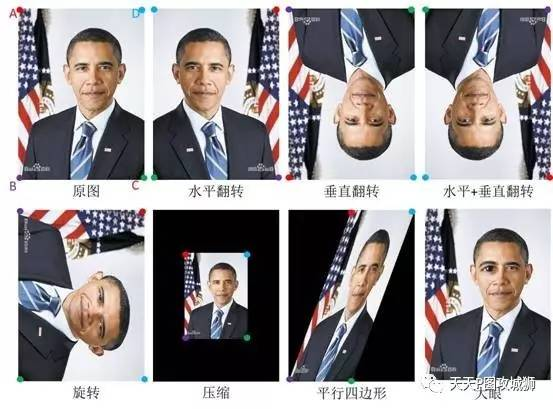# GPUImage的图像形变设计（简单形变部分)

GPUImage是iOS平台主流的GPU图像处理框架，能够非常方便地使用GPU对图像进行处理，包括：滤镜、分布统计等。#### 1. 翻转

vec2 newTex = vec2(1.0 – textureCoordinate.x, textureCoordinate.y); //代表水平翻转vec2 newTex = vec2(textureCoordinate.x, 1.0 – textureCoordinate.y); //代表垂直翻转vec2 newTex = vec2(1.0 – textureCoordinate.x, 1.0 - textureCoordinate.y); //代表水平+垂直翻转

#### 2. 旋转

vec2 newTex = vec2(textureCoordinate.y, 1.0 - textureCoordinate.x);  //代表向右旋转90度

### 3. 压缩

vec2 newTex = vec2(textureCoordinate.x * 2.0 – 0.5, textureCoordinate.y * 2.0 – 0.5);

#### 4. 变平行四边形

vec2 newTex = vec2(textureCoordinate.x * 2.0 + textureCoordinate.y – 1.0, textureCoordinate.y);

#### 5. 大眼

vec2 center = vec2(x0, y0);
vec2 newTex = textureCoordinate.xy;float dist = sqrt((center.x – newTex.x)* (center.x – newTex.x) + (center.y – newTex.y)* (center.y – newTex.y));if (dist < r){
float ratio = sqrt(dist / r);
float x1 = center.x – (center.x – newTex.x) * ratio;
float y1 = center.y – (center.y – newTex.y) * ratio;
newTex = vec2(x1, y1);
}#### 小结一下：

##### Part2：基于自定义vertices的图像形变设计//不使用默认的顶点//默认的vertices: {-1.0, -1.0, 1.0, -1.0, -1.0, 1.0, 1.0, 1.0}//glVertexAttribPointer(filterPositionAttribute, 2, GL_FLOAT, 0, 0, vertices);       GLfloat meshVertices = {1.0, -1.0, -1.0, -1.0, 1.0, 1.0, -1.0, 1.0};
glVertexAttribPointer(filterPositionAttribute, 2, GL_FLOAT, 0, 0, &meshVertices);

#### 小结一下：